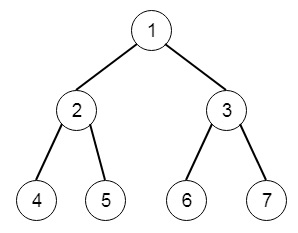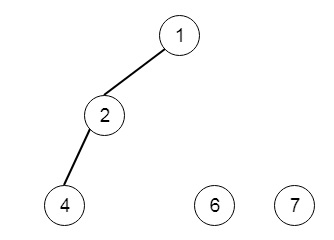# Delete Nodes And Return Forest in Python

Suppose we have the root of a binary tree, each node in the tree has a unique value. After removing all nodes with a value in to_delete, we are left with a forest. We have to find the roots of the trees in the remaining forest. So if the input is likeif the to_delete array is like [3,5], then the output will beTo solve this, we will follow these steps −

• Define an array res
• Define a method solve(), this will take node, to_delete array and a Boolean type info to which is telling that the node is root or not. The method will act like below −
• if node is null, then return null
• flag := true if value of node is in to_delete array
• if flag is false and is_root is true, then insert node into res
• left of node := solve(left of node, to_delete, flag)
• right of node := solve(right of node, to_delete, flag)
• return none when flag is set, otherwise false
• Call the solve() method from the main method like solve(node, to_delete, true)

Let us see the following implementation to get better understanding −

## Example

class Solution(object):
def delNodes(self, root, to_delete):
"""
:type root: TreeNode
:type to_delete: List[int]
:rtype: List[TreeNode]
"""
to_delete = set(to_delete)
self.res = []
self.solve(root,to_delete,True)
return self.res
def solve(self,node,to_delete,is_root):
if not node:
return None
flag = node.val in to_delete
if not flag and is_root:
self.res.append(node)
node.left = self.solve(node.left,to_delete,flag)
node.right = self.solve(node.right,to_delete,flag)
return None if flag else node

## Input

[1,2,3,4,5,6,7]
[3,5]

## Output

[[1,2,null,4],,]